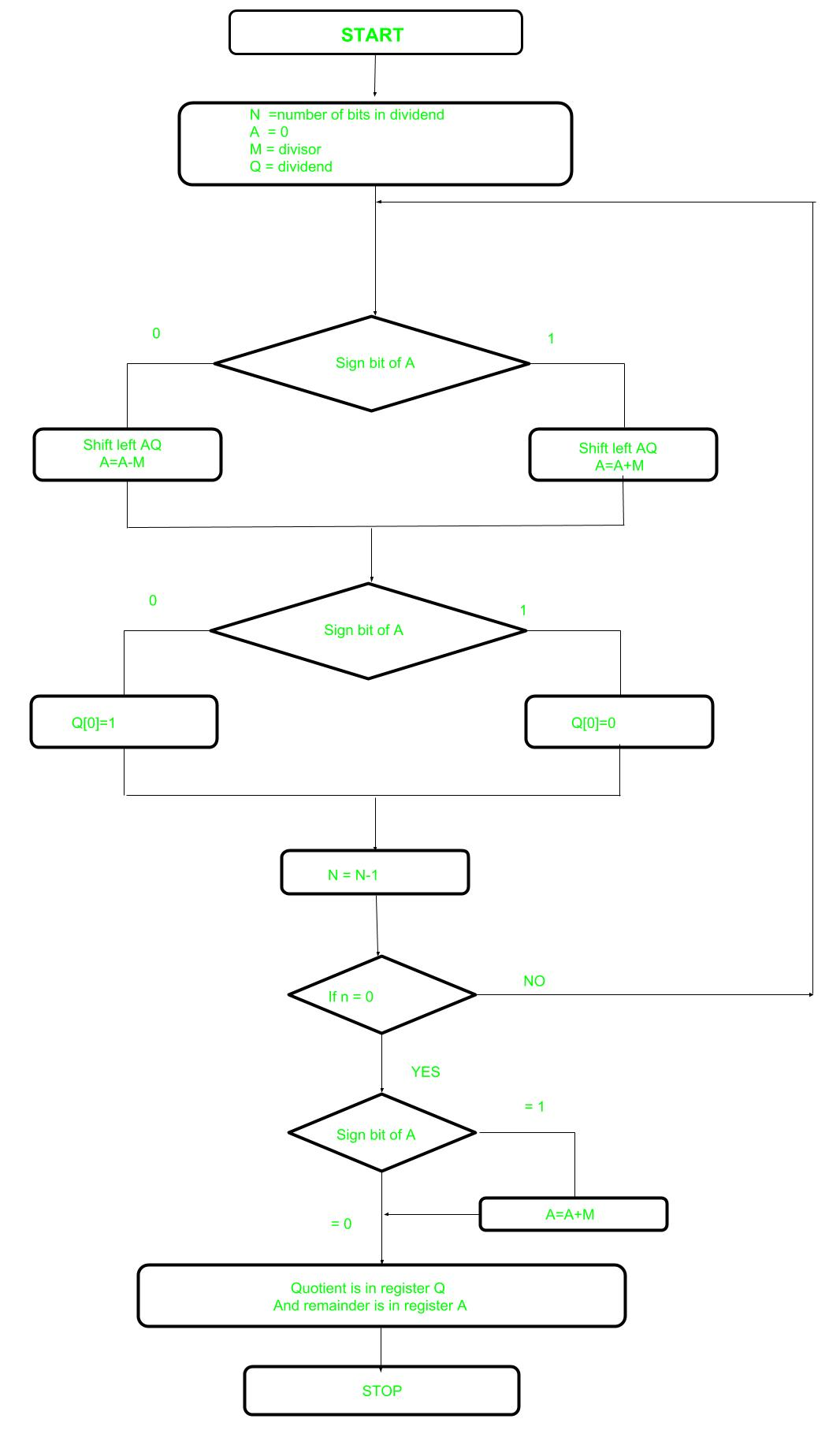# Non-Restoring Division For Unsigned Integer

• Difficulty Level : Easy
• Last Updated : 04 Oct, 2018

In earlier post Restoring Division learned about restoring division. Now, here perform Non-Restoring division, it is less complex than the restoring one because simpler operation are involved i.e. addition and subtraction, also now restoring step is performed. In the method, rely on the sign bit of the register which initially contain zero named as A.

Here is the flow chart given below.

Attention reader! Don’t stop learning now.  Practice GATE exam well before the actual exam with the subject-wise and overall quizzes available in GATE Test Series Course.

Learn all GATE CS concepts with Free Live Classes on our youtube channel.Let’s pick the step involved:

• Step-1: First the registers are initialized with corresponding values (Q = Dividend, M = Divisor, A = 0, n = number of bits in dividend)
• Step-2: Check the sign bit of register A
• Step-3: If it is 1 shift left content of AQ and perform A = A+M, otherwise shift left AQ and perform A = A-M (means add 2’s complement of M to A and store it to A)
• Step-4: Again the sign bit of register A
• Step-5: If sign bit is 1 Q become 0 otherwise Q become 1 (Q means least significant bit of register Q)
• Step-6: Decrements value of N by 1
• Step-7: If N is not equal to zero go to Step 2 otherwise go to next step
• Step-8: If sign bit of A is 1 then perform A = A+M
• Step-9: Register Q contain quotient and A contain remainder

Examples: Perform Non_Restoring Division for Unsigned Integer

```Dividend =11
Divisor  =3
-M =11101
```
NMAQAction
400011000001011Start
00001011_Left shift AQ
11110011_A=A-M
3111100110Q=0
11100110_Left shift AQ
11111110_A=A+M
2111111100Q=0
11111100_Left Shift AQ
00010100_A=A+M
1000101001Q=1
00101001_Left Shift AQ
00010001_A=A-M
0000100011Q=1
```Quotient  = 3 (Q)
Remainder = 2 (A)
```
My Personal Notes arrow_drop_up